# Meso Traffic Modeling¶

The main difference between the mesoscopic and microscopic approach is the use of discrete events to simulate vehicle movements through the network rather than the simulation of discrete time steps. With this exception, most of the modules and concepts in Aimsun Next are shared between meso and microscopic simulation. For example, the vehicle entrance process, traffic demand data, network geometry, vehicle data, and control plan are common to both modes.

## Vehicle Entrance Process¶

The traffic generation process determines the headway times for each vehicle. These headways are used to generate the vehicles that will enter using dynamic network loading. Vehicles enter into the network via origin centroids, where an origin centroid can be connected either to a section or to a node. The network structure for both situations is depicted in the screenshot below.

In both cases, vehicles enter virtual sections or turns and enter the real network when the node server considers that there is enough space in the network. All vehicles always enter the model through a virtual section or a virtual turn.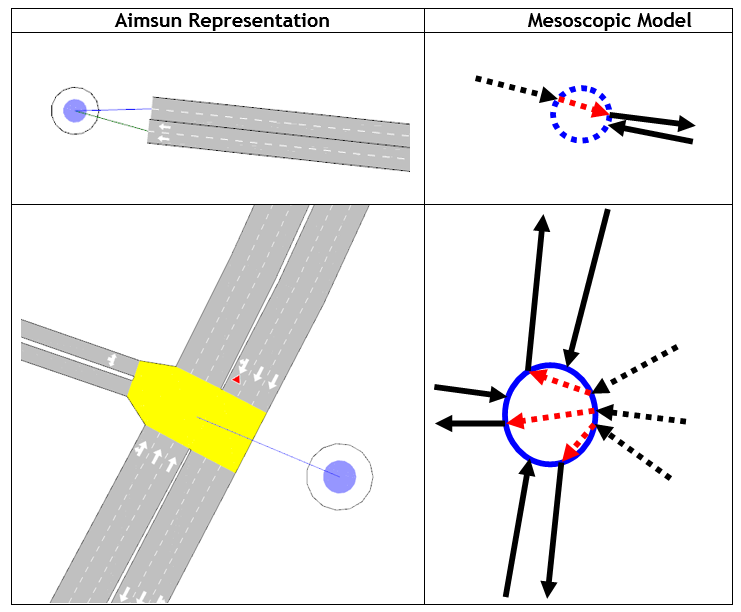## Modeling Vehicle Movement¶

Vehicle movement is divided into two models:

• Modeling vehicle movement in sections
• Car-following
• Lane-changing
• Modeling vehicle movement in nodes (Node Model)
• Modeling vehicle movement in turns
• Modeling vehicle movement from sections to turns
• Apply gap-acceptance model
• Apply lane-selection model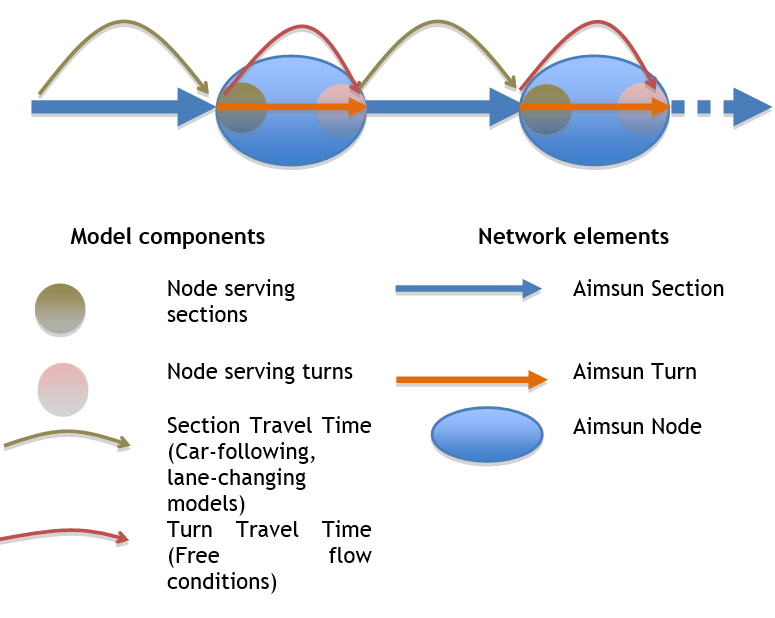Network elements are those objects that are present in all networks. In Aimsun Next there are sections and turns that connect two sections, and nodes, which are containers of turns. In the mesoscopic network representation, nodes are interpreted as mesoscopic nodes in the mesoscopic network representation.

In Aimsun Next, vehicles are assumed to move through sections and turns, so sections and turns become vehicle containers. A direct consequence of this is that all nodes are considered to be non-yellow boxes. The capacity SectionCapacity, in terms of the number of vehicles that can be contained at any time in a section, is calculated by using the jam density parameter and the length of the section.

SectionCapacity = JamDensity * Length * NumberLanes

where

• JamDensity is the Jam Density parameter defined in road sections
• Length is the section length, considering 3D coordinates
• NumberLanes is the total number of lanes in a section, considering on- and off-ramps

The turn capacity TurnCapacity is calculated in a similar way:

TurnCapacity = JamDensity * Length * NumberLanes

• JamDensity is the Jam Density parameter of the origin section
• Length is the turn length, considering 3D coordinates
• NumberLanes is the total number of destination lanes

Car-following and lane-changing models are applied to calculate the section travel time. This is the earliest time a vehicle can reach the end of the section, taking into account the current status of the section (number of vehicles in the section). Modeling of vehicle movements on sections in the mesoscopic simulator is based on the work of Mahut (1999a, 1999b, 2001).

The maximum capacity (veh/h) per lane can be calculated from three input parameters: speed, jam density, and reaction time. The speed is calculated using the vehicle's desired speed, the section speed limit,and the speed limit acceptance. The jam density is the jam density of the section and the reaction time is the reaction time of the vehicle, defined in the experiment, multiplied by the reaction-time factor.

#### Reaction Time Factor¶

The reaction-time factor can be set at both section and turn levels. The section reaction-time factor is used in the following situations:

• Meterings, when changing from red to green.
• Lane selection model to select the departure lane.
• Car-following model to calculate the intermediate section entrance times in the particular case of sections that are cut internally for the model calculations, for example when there are on/off-ramps or incidents.
• In the car-following model the reaction time of the vehicle leader is also used to calculate a minimum headway with this leader. In this calculation the section reaction-time factor is also applied.
• Queue stats, in order to calculate the queue statistics the vehicle reaction time is used to calculate the waiting time in queue. In this calculation the section reaction-time factor is applied.

The turn reaction-time factor is used in the following situations:

• Traffic lights, when changing from red to green.
• Car-following – when the vehicle is in the turn, the turn reaction-time factor is used to calculate the node-exit times of the other turns that have spatial conflict with the turn, and it's also used to calculate the entrance time when the vehicle is about to enter the next section.

## Node Model ¶

The Aimsun Next mesoscopic simulator is based on a node model that moves vehicles from one section to the next section of their path. This model contains four classes of actions that are calculated in all nodes:

• Serving sections. This calculates the next vehicle to enter the node. This is done by applying the yield model and by using the section-exit times calculated by the car-following and lane-changing models. Once a vehicle has been identified as the next vehicle to enter the node (exit section), all posterior vehicle exit times affected by this vehicle are updated.

By doing this we ensure that all delays produced by downstream congestion are propagated to upstream vehicles. The mesoscopic simulation is event-based, so there is an event related to this action or network-state change. The event concerns the next vehicle to enter the node from any of its input sections. This event is called a "node event from section".

• Processing vehicles' exit from section using the node event from section: When a node event from a section is processed, the actions are:
• Calculate vehicle-turn travel time. This calculation is done using the assumption that lane changes are not allowed under any circumstance inside nodes. Therefore, this travel time is always considered in free-flow conditions.
• Update the backmost vehicle
• Apply the lane-selection model. This is the model used by Aimsun Next to calculate the origin and destination lanes during the section movement. This uses the valid target lanes model.
• Once the origin and destination lanes are known, the vehicle travel time is calculated by applying the car-following and lane-changing models.
• Serving turns. This server calculates the next vehicle to leave the node. To get this vehicle, the exit turn time and the conditions of the vehicle's downstream section are taken into account. Once a vehicle has been selected, a new event called "node event from turn" is scheduled.

• Processing vehicles' exit from a node using the node event from turn.

• Move the previously identified vehicle to its next downstream section and queue this vehicle for its departure lane. Apply the lane-changing model to calculate the delay due to lane changing and calculate the earliest time that this vehicle will reach the end of the section.

## Yield Model¶

The yield model is used to model yield behaviors. In particular, the model is used when resolving node events in order to decide which of two vehicles in a conflict movement have priority. The following screenshots show some examples of conflict movements.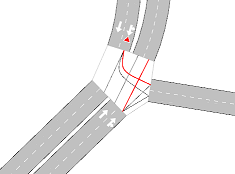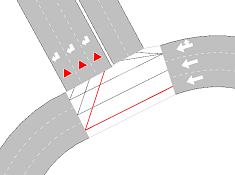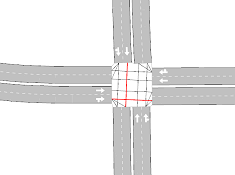The yield model is applied only in the first and second examples in the previous screenshots. In the third example, vehicles do not have any yield or stop signs, so the model is not applied and the first-in, first out (FIFO) rule is applied by using the corresponding vehicle demand times. Note that the vehicle demand time is the time at which the vehicle is ready to move to the next turn.

The general model is as illustrated below.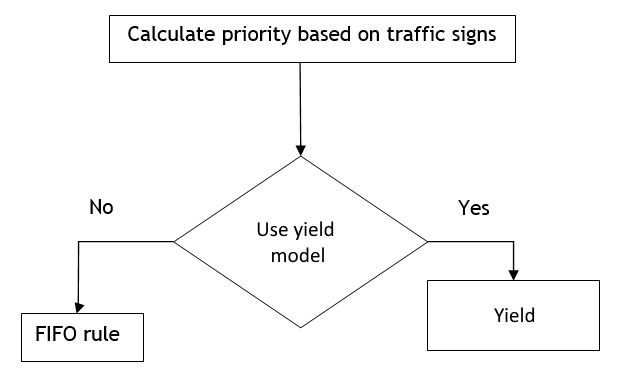The yield model used in the mesoscopic approach is a simplification of the yield model used by the microscopic simulator. The model takes into account the demand times of vehicles that are approaching the conflict movement. Demand times are used to calculate the gap required for a vehicle with a yield restriction to enter into the node. In the next screenshot, the red vehicle has to yield to the blue vehicle.

Let TD~R~ be the demand time of the red vehicle. This is the current time estimation for the red vehicle to enter into the node. Let TD~B~ be the demand time of the blue vehicle. This is the current time estimation to enter into the node with the conflict movement.

If there is no vehicle in the immediate input sections to the conflict node then the model looks for vehicles upstream until a maximum distance is reached. In the second screenshot below, Major section 1 is empty, so the model looks at its upstream section, which in this case is Major section 2. The value of TD~B~ now takes into account the demand time of the blue section in Major section 2 and then calculates the travel time of Major section 1, in free flow, to calculate the TD~B~. Only vehicles in the main stream with trajectories that are in conflict with the red vehicle are considered.

In this example, if the blue vehicle turns right instead of going straight ahead the red vehicle has no need to yield. The distance that the model looks upstream can be set in the road type or turn editor and is called Visibility along main stream: see the Road Type Editor or Turn Editor.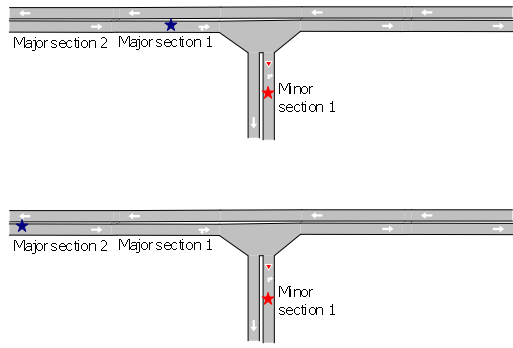Once TD~R~ and TD~B~ have been calculated, the gap is (TD~B~ - TD~R~). This is used to decide whether the red vehicle needs to yield or not. The waiting time of the red vehicle is used to calculate the maximum gap using the function in the next screenshot. This shows how the required gap changes as a function of a vehicle's waiting time. If the gap is in the blue shaded area then the red vehicle can pass through the node; otherwise it has to stop and cannot enter the node.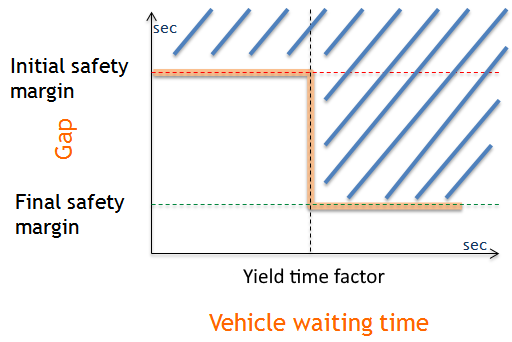The initial safety margin, final safety margin and yield time factor can be changed in the road type or in the turn parameters. Initially, the vehicle has to wait in the minor section until it has a gap greater than the initial safety margin. Once it has waited until the vehicle's maximum yield time multiplied by the yield time factor, the minimum gap required to make the movement is reduced to the final safety margin.

## Lane-Selection Model¶

This model is used to calculate the origin and destination lanes. As described in the node model, these calculations are made during the treatment of the “Node Event from Sections” event which occurs before the vehicle enters the turn.

The mesoscopic simulator calculates a default movement from all exit lanes at the beginning of the simulation. This means that from each lane in a section, there is a default next lane for each turn. For example in the Meso Look Ahead model ( see below) the red vehicle that is in its left most lane will have as its default next lane the left most lane from its next section.

To calculate the connectors inside the turn, the mesoscopic simulator uses the same internal heuristics used by the microscopic simulator. The next lane choice model for the mesoscopic simulator then uses two more heuristics to decide the next lane movement:

• Using next section status.
• Using valid target lanes model.

From the default lane choice, the mesoscopic simulator looks for the best entrance lane from all turn destination lanes. This is a major difference between the microscopic and mesoscopic simulation as the microscopic simulator only looks for a subset of all destination lanes.

To change the default lane choice, Aimsun Next takes into account the density of all lanes and the cost of changing the default lane choice. It also considers the turn connectors and initially selects the connector with the minimum distance. The example in the next screenshot shows where the turn (537 to 605) is fully connected from all origin lanes to all destination lanes, and lane 1 is connected to lanes [1,2,3] and lane 2 is connected to lanes [4,5]. By default the next entrance lane is lane 3 because it is the connector with the minimum distance. The distance is calculated using a straight line from point A to point B.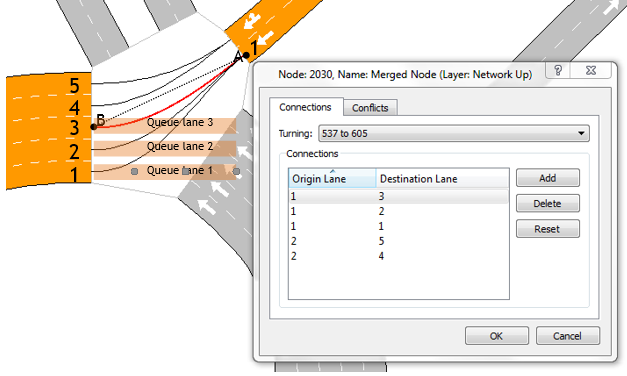For each turn there is a queue for each destination lane. The capacity of these queues is the jam density of the origin section multiplied by the turn length.

If queue for lane 3 is full then the simulator will try with lane 2 selecting lane choices in order of increasing distance/.

Alternately, to change the default lane choice, apply the valid target lanes model. The valid target lanes model implemented in the mesoscopic simulator is using the same approach used in the microscopic simulation.

Until now, the assumption has been that a vehicle only considers its next two turns.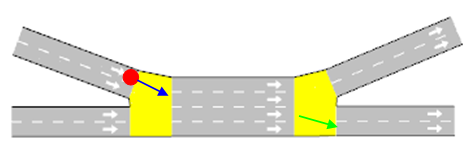In the example above, the red vehicle is using the blue and green turns to determine the origin and exit lanes for the final section. This means that the lane-selection model is based on the two turns preceding the final section.

In some situations, like in urban networks where there are short sections, or on freeways where weaving sections are relatively short, vehicles will take downstream turn movements into account in their lane-detection decision.

The default lane, when there is a restricted range of entrance lanes due to a turn conflict, is the closest lane to the original default lane.

As illustrated, the range of possible entrance lanes to the final section (in green) can differ depending on the range of entrance lanes of the downstream section.

When there are multiple possible lanes. a penalty is calculated for each lane. The penalty function is based on the number of lane changes needed to reach the target lane and the densities observed on each lane of the section. There are two optional penalties that can be set for each section:

• Penalize shared lanes. When this option is ticked, a penalty is added for those lanes that belong to different turns.

• Take into account fast/slow lanes. This option favors fast vehicle types using fast lanes and slow vehicle types using slow lanes.

Both penalties are the same as those that are used to penalize the number of lane changes. Once a penalty is calculated for each lane, the lane with the minimum penalty is selected. If there are multiple lanes with the same minimum penalty the lane is selected at random.

## Mesoscopic Simulation: Random Seeds¶

To have more control over the randomness and variability of the mesoscopic simulator in DUE and SRC simulations, five different seeds can be set independently. This enables the simulation of different replications with, for example, the generation of different private vehicles but with the same transit vehicles. These seeds can be set in the Attributes tab of the result editor.

• Transit: This seed is used in the generation of the transit vehicles. It controls the vehicle generation times and the stopping times at bus stops.
• Traffic Management: This seed is used by traffic-management actions.
• Vehicle Assignment: This seed is used by the vehicle-assignment process to select the path for each vehicle.
• Vehicle Generation: This seed is used by the vehicle-generation process for private cars to assign vehicle parameters. The vehicle-generation times, as vehicles are released into the simulation, depend on the general random seed.

For each seed, if the value is set to 0, then the random number is taken from the general random seed that is defined in the main tab of the Result editor.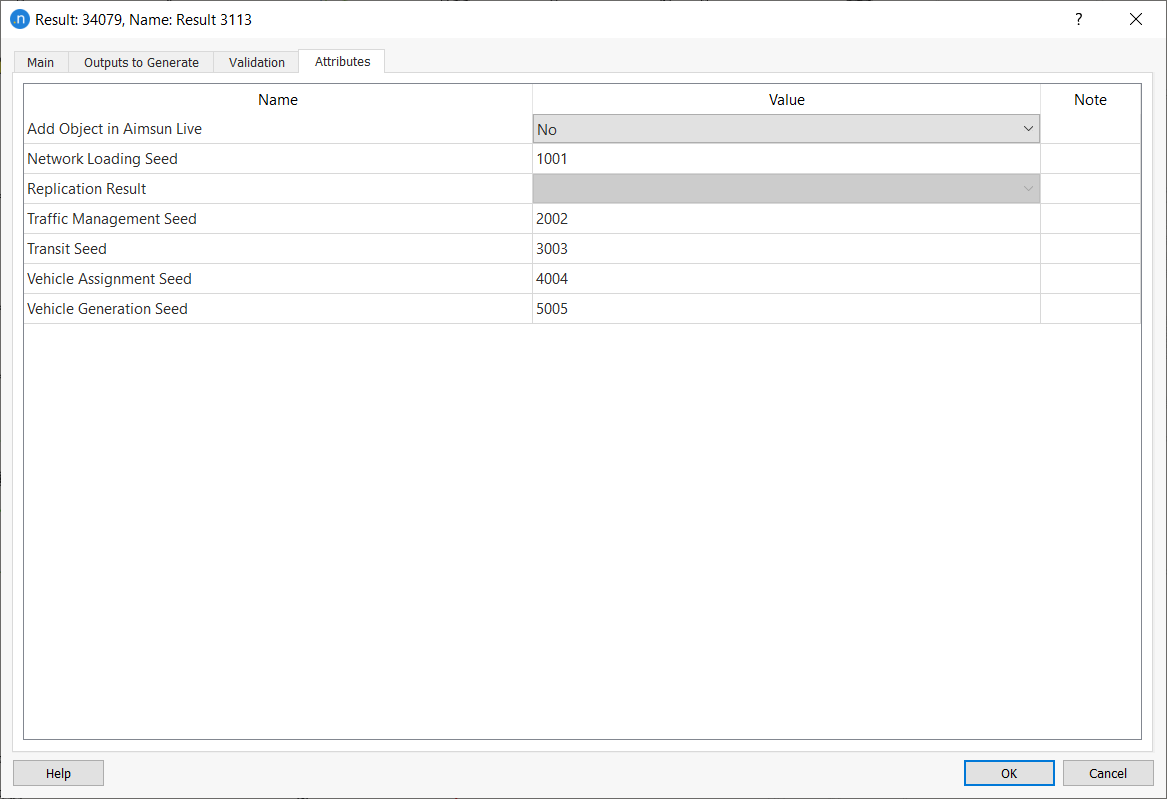## Mesoscopic On-Ramp Behavior¶

To model on-ramps, the mesoscopic simulation cuts the section with the on-ramp into different segments. For example, the following road section has two internal segments; the first segment has three lanes and a length of 100 meters and the second segment has two lanes and is 300 meters long.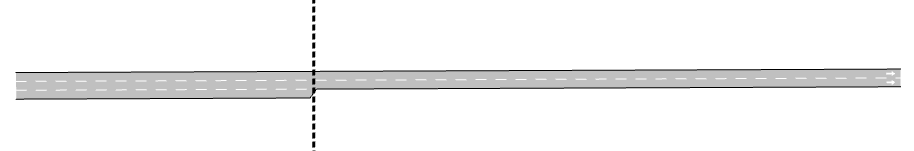Internally, two sections are created as shown below.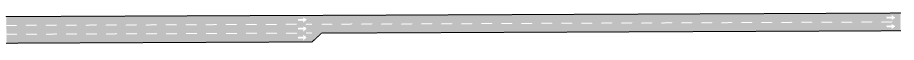Here, instead of an on-ramp being part of the section, the on-ramp is implemented as two different sections connected with a node. The behavior will be different because in the second case there is a node and a turn. By definition, all turns have a minimum capacity of one vehicle, so in the second case vehicles will move from the first section on the left to the turn, and then from the turn to the next section on the right. In general though, it is more convenient to model on-ramps by coding them as in the first example.

There are two parameters that you can use to calibrate the behavior of the mesoscopic on-ramp model: Cooperation Gap and Merging Gap.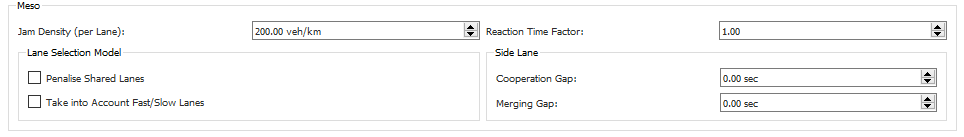• Cooperation Gap: It is the amount of time (in seconds) that the mainline vehicles will force to have as headway whenever side-lane (on-ramp) merging vehicles are present. This is to facilitate the incorporation of traffic. The only vehicle that will be delayed is the vehicle on the first mainline lane adjacent to the ramp.

• Merging Gap: It is the minimum gap that vehicles in the side lane (on-ramp) look for in order to merge into the mainline.